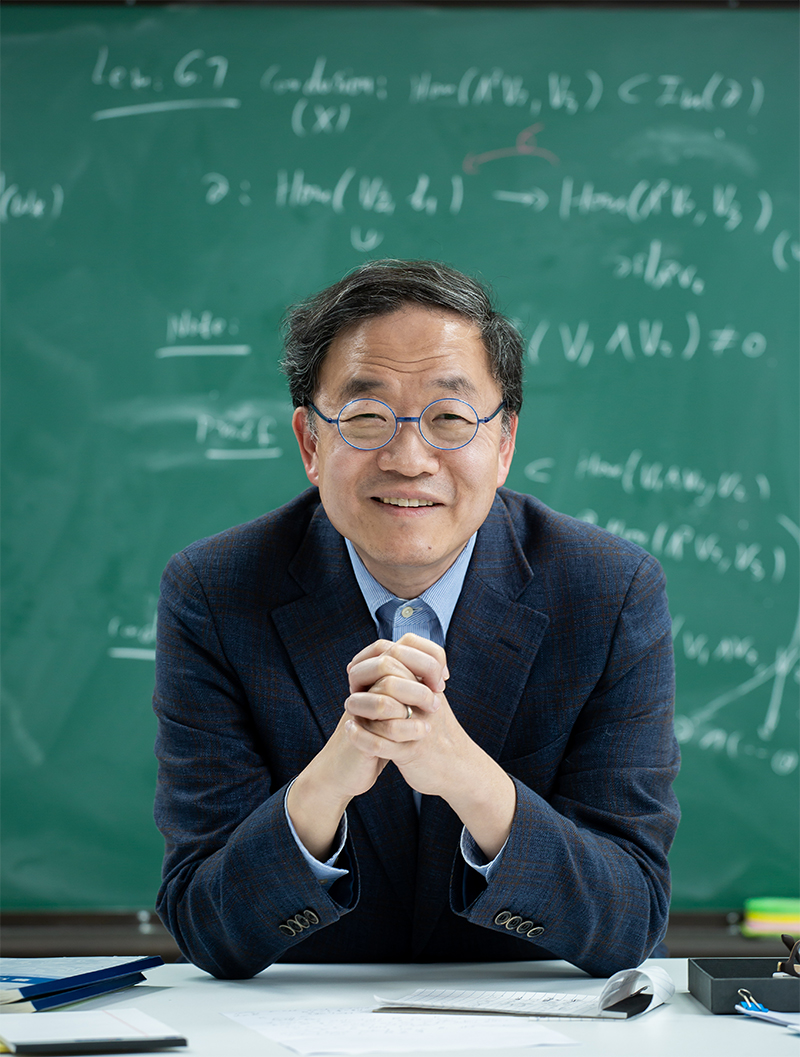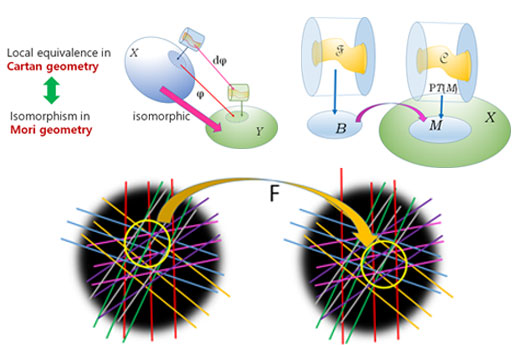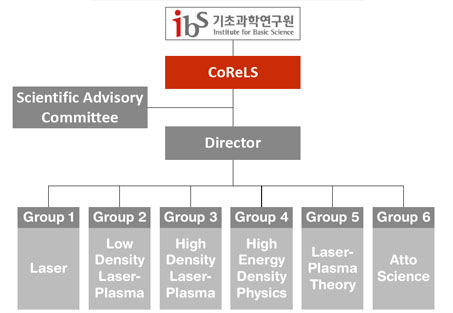DirectorHWANG Jun Muk

Attacking challenging problems through geometry over complex numbers

Contact Info

Tel. +82-42-878-8180

IBS Center for Complex Geometry,
B275, 55 EXPO-ro, Doryong-dong,
Yuseong-gu, Daejeon (34126)

#### Major Publications

• - An application of Cartan’s equivalence method to Hirschowtiz’s conjecture on the formal principle
• - Webs of Lagrangian tori in projective symplectic manifolds
• - Classification of non-degenerate projective varieties with non-zero prolongation and application to target rigidity
• - Base manifolds for fibrations of projective irreducible symplectic manifolds
##### DirectorDirector HWANG Jun Muk

Dr. HWANG is the director of the Center for Complex Geometry, established in September 2020.
Dr. HWANG had B.A. in 1986 from Physics department of Seoul National University and Ph. D. in 1993 from Mathematics department of Harvard University. He had worked in University of Notre Dame and Seoul National University before becoming a professor at Korea Institute for Advanced Study in 1999. He was awarded Korea Science Prize in 2001 and Ho-Am Prize in 2009.

##### IntroductionAttacking challenging problems on systems of polynomial equations and differential equations by fusing ideas and tools from algebraic geometry, differential geometry and complex analysis of several variables.

• - Developing methods of differential geometry to study problems in algebraic geometry.
• - Attacking old unsolved problems and proposing new problems in the intersection of algebra and analysis over complex numbers.
• - Studying symmetries and invariants of systems of differential equations over complex numbers.
##### Main research activities

Since its emergence as one of the core areas of mathematics in early 19th century, the development of complex analysis has been intricately related to geometry. In the 20th century, the development of complex analysis of several variables intensified its interaction with algebraic geometry and differential geometry. Nowadays it is often hard to distinguish whether a problem or a method in this area belongs to algebraic geometry, differential geometry or complex analysis of several variables. The fusion of these areas of algebra, geometry and analysis over complex numbers is Complex Geometry.

The research at IBS Center for Complex Geometry is centered around the interaction of algebraic geometry and differential geometry. This interaction is much more natural and transparent when we work with complex numbers than real numbers. We aim to develop novel methodology to attack challenging problems on systems of polynomial equations and differential equations. The geometric nature of systems of equations is reflected in their symmetries and invariants so that the main techniques we employ involve Lie theory as well.

At Center for Complex Geometry, mathematicians from these diverse areas interact to learn different ideas from one another and collaborate to develop new methods to attack unsolved problems in mathematics.

##### Organization##### Main research results
• 1.5 PW Laser Beamline 4PW improves performance - Extreme laser field attainmnet
(Optics Express, 2019)
• Propose a powerful gamma-ray generation mechanism by non-linear compton scattering at targets with critical and solid densities
(New. J Phys, 2019)
• Discovery of polarized radiation generated by a violent field phenomenon
(Nature Photonics, 2018)
• Development of OPCPA amplifier; changeable spectrum
(Optics Express, 2018)
• Resolving Multiple Molcular Orbitals Using Two-Dimensional High-Harmonic Spectroscopy
(Physical Review Letters , 2015)
• Transition of Proton Energy Scaling Using an Ultrathin Target Irradiated by Linearly Polarized Femtosecond Laser Pulses
(Physical Review Letters, 2013)
• Enhancement of Electron Energy to the Multi-Gev Regime by a Dual-Stage Laser-Wakefield Accelerator Pumped by Petawatt Laser
(Physical Review Letters, 2013)
##### Personnel
Total 71 64(Male), 7(Female) 64(Korean), 7(International)
##### Position

As of October. 2019

Research